Test: Probability & Statistics

# Test: Probability & Statistics

Test Description

## 20 Questions MCQ Test GATE Electrical Engineering (EE) 2023 Mock Test Series | Test: Probability & Statistics

Test: Probability & Statistics for Electronics and Communication Engineering (ECE) 2023 is part of GATE Electrical Engineering (EE) 2023 Mock Test Series preparation. The Test: Probability & Statistics questions and answers have been prepared according to the Electronics and Communication Engineering (ECE) exam syllabus.The Test: Probability & Statistics MCQs are made for Electronics and Communication Engineering (ECE) 2023 Exam. Find important definitions, questions, notes, meanings, examples, exercises, MCQs and online tests for Test: Probability & Statistics below.
Solutions of Test: Probability & Statistics questions in English are available as part of our GATE Electrical Engineering (EE) 2023 Mock Test Series for Electronics and Communication Engineering (ECE) & Test: Probability & Statistics solutions in Hindi for GATE Electrical Engineering (EE) 2023 Mock Test Series course. Download more important topics, notes, lectures and mock test series for Electronics and Communication Engineering (ECE) Exam by signing up for free. Attempt Test: Probability & Statistics | 20 questions in 60 minutes | Mock test for Electronics and Communication Engineering (ECE) preparation | Free important questions MCQ to study GATE Electrical Engineering (EE) 2023 Mock Test Series for Electronics and Communication Engineering (ECE) Exam | Download free PDF with solutions
 1 Crore+ students have signed up on EduRev. Have you?
Test: Probability & Statistics - Question 1

### If the arithmetic mean of two numbers is 10 and their geometric mean is 8, the numbers are

Detailed Solution for Test: Probability & Statistics - Question 1

Let the numbers be a and b Then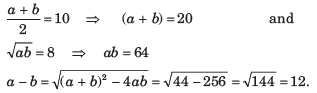Solving a + b = 20 and a - b = 12 we get a  = 16 and b = 4.

Test: Probability & Statistics - Question 2

### The median of 0, 2, 2, 2, -3, 5, -1, 5, 5, -3, 6, 6, 5, 6 is

Detailed Solution for Test: Probability & Statistics - Question 2

Observations in ascending order are -3, -3, -1, 0, 2, 2, 2, 5, 5, 5, 5 6, 6, 6
Number of observations is 14, which is even.Test: Probability & Statistics - Question 3

### Consider the following table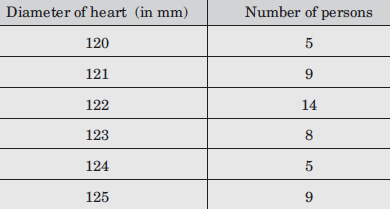The median of the above frequency distribution is

Detailed Solution for Test: Probability & Statistics - Question 3

The given Table may be presented as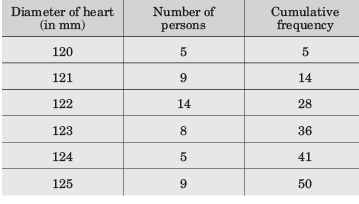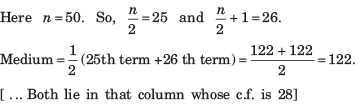Test: Probability & Statistics - Question 4

The mode of the following frequency distribution, is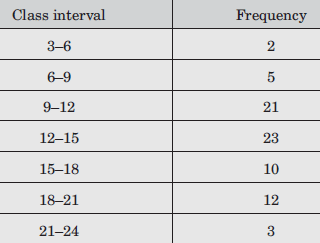Detailed Solution for Test: Probability & Statistics - Question 4

Maximum frequency is 23. So, modal class is 12–15.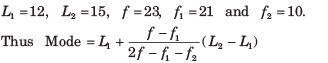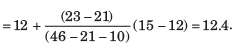Test: Probability & Statistics - Question 5

The mean-deviation of the data 3, 5, 6, 7, 8, 10, 11, 14 is

Detailed Solution for Test: Probability & Statistics - Question 5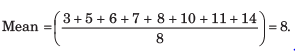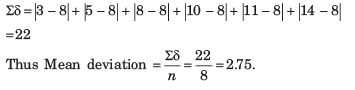Test: Probability & Statistics - Question 6

The mean deviation of the following distribution is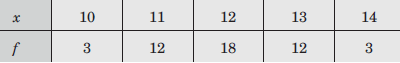Detailed Solution for Test: Probability & Statistics - Question 6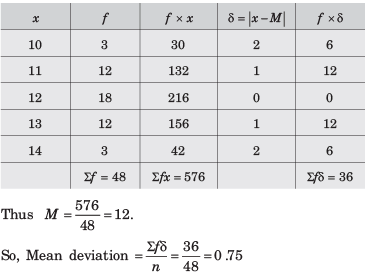Test: Probability & Statistics - Question 7

The standard deviation for the data 7, 9, 11, 13, 15 is

Detailed Solution for Test: Probability & Statistics - Question 7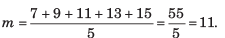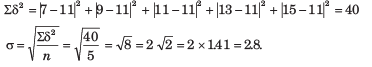Test: Probability & Statistics - Question 8

The standard deviation of 6, 8, 10, 12, 14 is

Detailed Solution for Test: Probability & Statistics - Question 8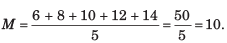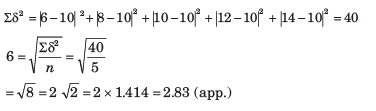Test: Probability & Statistics - Question 9

The probability that an event A occurs in one trial of an experiment is 0.4. Three independent trials of experiment are performed. The probability that A occurs at least once is

Detailed Solution for Test: Probability & Statistics - Question 9

Here p  = 0.4, q = 0.6 and n = 3.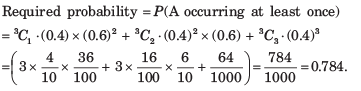Test: Probability & Statistics - Question 10

Eight coins are tossed simultaneously. The probability of getting at least 6 heads is

Detailed Solution for Test: Probability & Statistics - Question 10

p = 1/2, q = 1/2, n = 8. Required probability  = P (6 heads or 7 heads or 8 heads)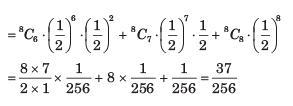Test: Probability & Statistics - Question 11

The ranks obtained by 10 students in Mathematics and Physics in a class test are as follows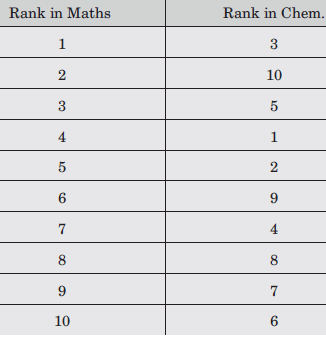The coefficient of correlation between their ranks is

Detailed Solution for Test: Probability & Statistics - Question 11

Di = -2, -8, -2, 3, 3, -3, 3, 0, 2, 4.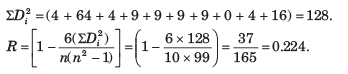Test: Probability & Statistics - Question 12

If ∑ xi = 24, ∑yi = 44, ∑ xiyi =  306,  ∑ xi2 =164 ,∑yi2 = 574 and n = 4 then the regression coefficient bxy  is equal to

Detailed Solution for Test: Probability & Statistics - Question 12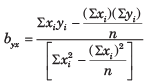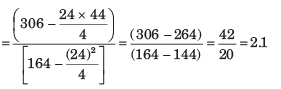Test: Probability & Statistics - Question 13

If ∑ xi = 30, ∑yi = 42, ∑ xiyi =  199 , ∑ xi2 =184 ,∑yi2 = 318 and n = 6 then the regression coefficient bxy  is equal to

Detailed Solution for Test: Probability & Statistics - Question 13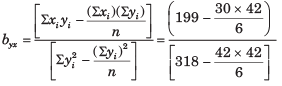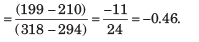Test: Probability & Statistics - Question 14

Let r be the correlation coefficient between x and y and bxy ,byx be the regression coefficients of y on x and x on y respectively then

Detailed Solution for Test: Probability & Statistics - Question 14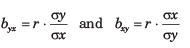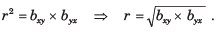Test: Probability & Statistics - Question 15

Which one of the following is a true statement

Detailed Solution for Test: Probability & Statistics - Question 15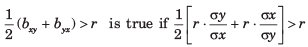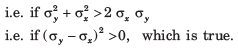Test: Probability & Statistics - Question 16

If byx  = 1.6 and bxy = 0.4 and θ is the angle between two regression lines, then tan θ is equal to

Detailed Solution for Test: Probability & Statistics - Question 16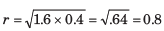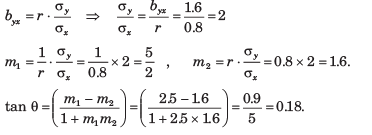Test: Probability & Statistics - Question 17

The equations of the two lines of regression are : 4x + 3y + 7 = 0 and 3x + 4y + 8  = 0. The correlation coefficient between x and y is

Detailed Solution for Test: Probability & Statistics - Question 17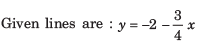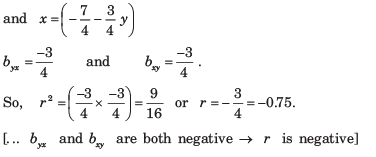Test: Probability & Statistics - Question 18

If cov(X, Y) = 10, var (X) = 6.25 and var(Y)  = 31.36, then ρ(X,Y) is

Detailed Solution for Test: Probability & Statistics - Question 18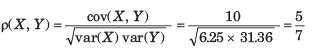Test: Probability & Statistics - Question 19

If ∑x = ∑y = 15, ∑x2 = ∑y2 = 49, ∑xy = 44 and n = 5, then bxy = ?

Detailed Solution for Test: Probability & Statistics - Question 19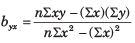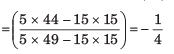Test: Probability & Statistics - Question 20

If ∑x = 125, ∑y = 100 , ∑x2 = 1650, ∑y2 = 1500 , ∑xy = 50 and n = 25, then the line of regression of x on y is

Detailed Solution for Test: Probability & Statistics - Question 20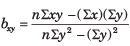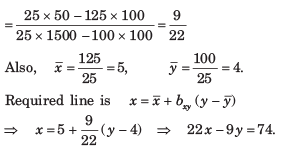## GATE Electrical Engineering (EE) 2023 Mock Test Series

22 docs|274 tests
Information about Test: Probability & Statistics Page
In this test you can find the Exam questions for Test: Probability & Statistics solved & explained in the simplest way possible. Besides giving Questions and answers for Test: Probability & Statistics, EduRev gives you an ample number of Online tests for practice

## GATE Electrical Engineering (EE) 2023 Mock Test Series

22 docs|274 tests(Scan QR code)